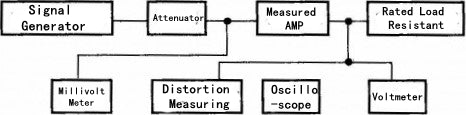### How to measure and calculate output power of power amplifier

0 comments / Posted on by Vincent Zhang

# How to measure and calculate output power of power amplifier

There are three output powers commonly used, rated output power, maximum output power and music power. Here is tube amplifier as an example.

1. Rated output power (RMS): When tube power amplifier frequency characteristic and harmonic distortion coefficient can reach the specified technical specifications (generally tube power amplifier distortion should be less than 1%), power amplifier can work continuously for a long time safe continuous sinusoidal signal power.
2. Maximum output power (PM): it can meet the basic parameters that Maximum output power of the power amplifier at the rated load resistance.
3. Music Power (MPO): the instantaneous power value that power amplifier can output when Input a large dynamic music signal and keeping that there is not voltage drop of amplifier power supply. This MPO power is typically 4 or 6 times RMS power.

The output power of tube amplifier is measured by output a 1 kHz sine wave signal from audio signal generator to input to power amplifier to be measured through the attenuator, and send to input end of the measured channel is placed at the maximum position, and other channels are placed at the minimum position, and its tone control is placed at normal position as shown. Then connect a distortion measuring instrument to output terminal of rated load, increase input signal voltage, make the harmonic distortion coefficient of output voltage reach the corresponding specified value, and finally measure the output power under normal operation and continuous signal.

Output power measurement method block diagramThe output power is calculated as: P=U2/RL.

If the power amplifier is placed under an 8 ohm load and its output signal voltage is 20v and the distortion factor is 1%, so its rated output power (RMS) of the amplifier is

P= U2/ RL =202/8=50W

In addition, in order to simplify the method of output power of tube power amplifier measurement, we can use the method shown in the figure below to make measurements. The specific method is, placing the measured work in a normal working state, the volume potentiometer is placed at the maximum position, and the music signal output form CD player or the record player or the signal of 1 kHz signal generator self-made is input to the input end of the measured power amplifier, is connected to an 8 ohm speaker or an 8 ohm load resistor at output end of tube power amplifier, and the dynamic voltage across the load is measured by an AC voltmeter or a multimeter AC 20V gear. The average of measured value is its output voltage.If a tube power amplifier is placed at both ends of the speaker load and the output AC voltage is measured as 10v, the estimated power of its power amplifier is

P= U2/ RL = 102/8=12W